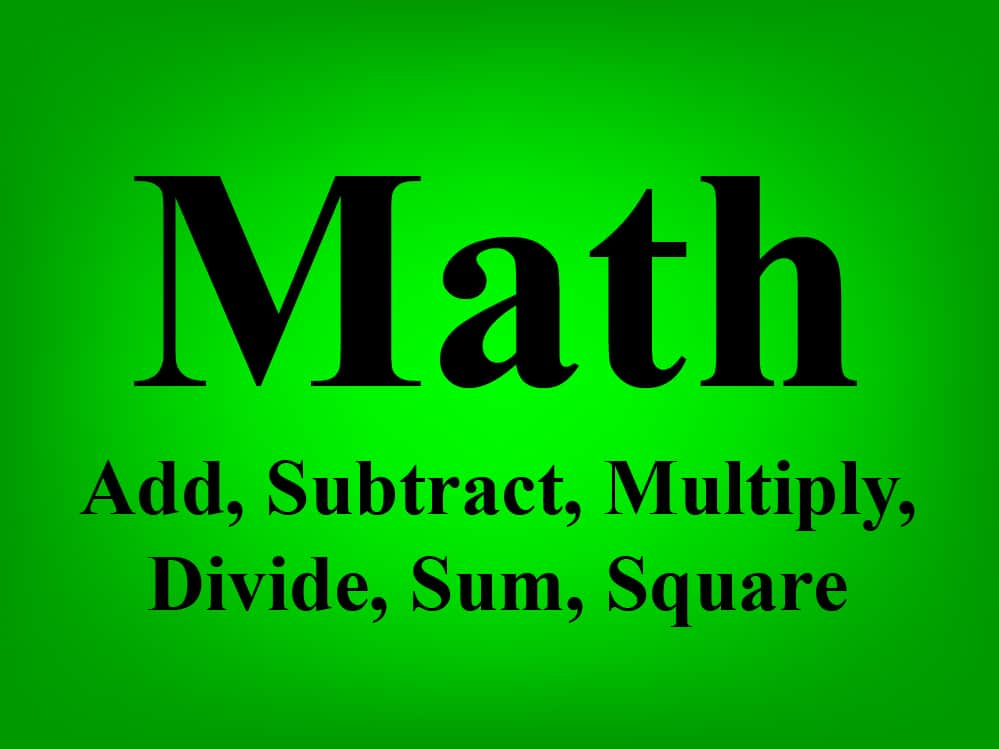# How much means add or subtract

79 views
how much means add or subtract

Addition means giving more to the value, such as adding the number 2 to the number 3 so that the result is 5.
And subtraction means taking a number from the original value to reduce the amount, such as: Subtracting the number 2 from the number 3 so that the final result is one.by (60.5k points) edited

18 views
18 views
91 views